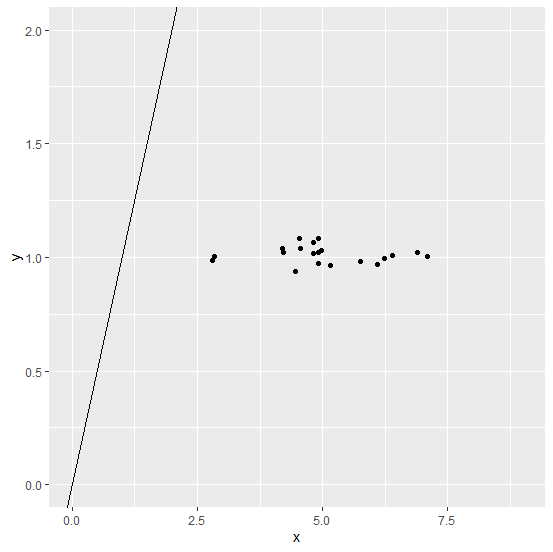# How to create a scatterplot with regression line using ggplot2 with 0 intercept and slope equals to 1 in R?

To create a regression line with 0 intercept and slope equals to 1 using ggplot2, we can use geom_abline function but we need to pass the appropriate limits for the x axis and y axis values. For example, if we have two columns x and y in a data frame df and both have ranges starting from -1 to 1 then the scatterplot with regression line with 0 intercept and slope equals to 1 can be created as −

ggplot(df,aes(x,y))+geom_point()+geom_abline()+lims(x=c(-1,1),y=c(-1,1))

## Example

Consider the below data frame −

Live Demo

x<-rnorm(20,5,1.12)
y<-rnorm(20,1,0.035)
df<-data.frame(x,y)
df

## Output

      x       y
1 4.553620 1.0369370
2 4.969202 1.0302152
3 2.794787 0.9859984
4 4.811202 1.0151066
5 2.834154 1.0035345
6 4.811507 1.0662929
7 4.542179 1.0820936
8 5.752000 0.9822227
9 6.396348 1.0068282
10 4.213624 1.0193361
11 7.096180 1.0021550
12 4.909697 1.0823344
13 4.205709 1.0407263
14 4.462543 0.9380729
15 6.103617 0.9695582
16 5.154046 0.9623501
17 4.913657 0.9750284
18 4.924041 1.0226467
19 6.230603 0.9971666
20 6.889095 1.0211787

Loading ggplot2 package and creating scatterplot with regression line having 0 intercept and slope equals to 1 −

## Example

library(ggplot2)
ggplot(df,aes(x,y))+geom_point()+geom_abline()+lims(x=c(0,9),y=c(0,2))

## Output